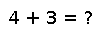# size

size(A, [dim...])

Returns a tuple containing the dimensions of `A`. Optionally you can specify the dimension(s) you want the length of, and get the length of that dimension, or a tuple of the lengths of dimensions you asked for.:

``````julia> A = rand(2,3,4);

julia> size(A, 2)
3

julia> size(A,3,2)
(4,3)``````

## Examples

``````julia> foo = [1,2,3];
julia> size(foo)
(3,)
julia> bar = Array(Int8, 3, 4);
julia> size(bar)
(3,4)
julia> size(bar, 1)
3``````

Checking you are not a robot: